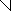# MINLPLib

### A Library of Mixed-Integer and Continuous Nonlinear Programming Instances

#### Instance chem

 Formatsⓘ ams gms mod nl osil py Primal Bounds (infeas ≤ 1e-08)ⓘ -47.70651483 p1 ( gdx sol ) (infeas: 4e-16) Other points (infeas > 1e-08)ⓘ Dual Boundsⓘ -47.70651533 (ANTIGONE)-47.70651488 (BARON)-47.70655845 (COUENNE)-47.70654626 (LINDO)-47.70652115 (SCIP) Referencesⓘ Bracken, Jerome and McCormick, Garth P, Chapter 5. In Bracken, Jerome and McCormick, Garth P, Selected Applications of Nonlinear Programming, John Wiley and Sons, New York, 1968, 48-49. Sourceⓘ GAMS Model Library model chem Applicationⓘ Chemical Equilibrium Added to libraryⓘ 31 Jul 2001 Problem typeⓘ NLP #Variablesⓘ 11 #Binary Variablesⓘ 0 #Integer Variablesⓘ 0 #Nonlinear Variablesⓘ 11 #Nonlinear Binary Variablesⓘ 0 #Nonlinear Integer Variablesⓘ 0 Objective Senseⓘ min Objective typeⓘ nonlinear Objective curvatureⓘ nonconcave #Nonzeros in Objectiveⓘ 11 #Nonlinear Nonzeros in Objectiveⓘ 11 #Constraintsⓘ 4 #Linear Constraintsⓘ 4 #Quadratic Constraintsⓘ 0 #Polynomial Constraintsⓘ 0 #Signomial Constraintsⓘ 0 #General Nonlinear Constraintsⓘ 0 Operands in Gen. Nonlin. Functionsⓘ div log mul Constraints curvatureⓘ linear #Nonzeros in Jacobianⓘ 25 #Nonlinear Nonzeros in Jacobianⓘ 0 #Nonzeros in (Upper-Left) Hessian of Lagrangianⓘ 31 #Nonzeros in Diagonal of Hessian of Lagrangianⓘ 11 #Blocks in Hessian of Lagrangianⓘ 1 Minimal blocksize in Hessian of Lagrangianⓘ 11 Maximal blocksize in Hessian of Lagrangianⓘ 11 Average blocksize in Hessian of Lagrangianⓘ 11.0 #Semicontinuitiesⓘ 0 #Nonlinear Semicontinuitiesⓘ 0 #SOS type 1ⓘ 0 #SOS type 2ⓘ 0 Minimal coefficientⓘ 1.0000e+00 Maximal coefficientⓘ 3.4021e+01 Infeasibility of initial pointⓘ 1.993 Sparsity JacobianⓘSparsity Hessian of Lagrangianⓘ```\$offlisting
*
*  Equation counts
*      Total        E        G        L        N        X        C        B
*          5        5        0        0        0        0        0        0
*
*  Variable counts
*                   x        b        i      s1s      s2s       sc       si
*      Total     cont   binary  integer     sos1     sos2    scont     sint
*         12       12        0        0        0        0        0        0
*  FX      0
*
*  Nonzero counts
*      Total    const       NL      DLL
*         37       26       11        0
*
*  Solve m using NLP minimizing objvar;

Variables  x1,x2,x3,x4,x5,x6,x7,x8,x9,x10,x11,objvar;

Equations  e1,e2,e3,e4,e5;

e1..    x1 + 2*x2 + 2*x3 + x6 + x10 =E= 2;

e2..    x4 + 2*x5 + x6 + x7 =E= 1;

e3..    x3 + x7 + x8 + 2*x9 + x10 =E= 1;

e4.. -((-6.05576803624071 + log(x1/x11))*x1 + (-17.1307680362407 + log(x2/x11))
*x2 + (-34.0207680362407 + log(x3/x11))*x3 + (-5.88076803624071 + log(x4/
x11))*x4 + (-24.6877680362407 + log(x5/x11))*x5 + (-14.9527680362407 +
log(x6/x11))*x6 + (-24.0667680362407 + log(x7/x11))*x7 + (-
10.6747680362407 + log(x8/x11))*x8 + (-26.6287680362407 + log(x9/x11))*x9
+ (-22.1447680362407 + log(x10/x11))*x10) + objvar =E= 0;

e5..  - x1 - x2 - x3 - x4 - x5 - x6 - x7 - x8 - x9 - x10 + x11 =E= 0;

* set non-default bounds
x1.lo = 0.001;
x2.lo = 0.001;
x3.lo = 0.001;
x4.lo = 0.001;
x5.lo = 0.001;
x6.lo = 0.001;
x7.lo = 0.001;
x8.lo = 0.001;
x9.lo = 0.001;
x10.lo = 0.001;
x11.lo = 0.01;

Model m / all /;

m.limrow=0; m.limcol=0;
m.tolproj=0.0;

\$if NOT '%gams.u1%' == '' \$include '%gams.u1%'

\$if not set NLP \$set NLP NLP
Solve m using %NLP% minimizing objvar;

```

Last updated: 2023-08-16 Git hash: 2519540e